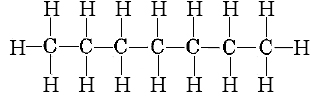# 15.12: Fossil Fuels and the Energy Crisis

$$\newcommand{\vecs}{\overset { \rightharpoonup} {\mathbf{#1}} }$$ $$\newcommand{\vecd}{\overset{-\!-\!\rightharpoonup}{\vphantom{a}\smash {#1}}}$$$$\newcommand{\id}{\mathrm{id}}$$ $$\newcommand{\Span}{\mathrm{span}}$$ $$\newcommand{\kernel}{\mathrm{null}\,}$$ $$\newcommand{\range}{\mathrm{range}\,}$$ $$\newcommand{\RealPart}{\mathrm{Re}}$$ $$\newcommand{\ImaginaryPart}{\mathrm{Im}}$$ $$\newcommand{\Argument}{\mathrm{Arg}}$$ $$\newcommand{\norm}{\| #1 \|}$$ $$\newcommand{\inner}{\langle #1, #2 \rangle}$$ $$\newcommand{\Span}{\mathrm{span}}$$ $$\newcommand{\id}{\mathrm{id}}$$ $$\newcommand{\Span}{\mathrm{span}}$$ $$\newcommand{\kernel}{\mathrm{null}\,}$$ $$\newcommand{\range}{\mathrm{range}\,}$$ $$\newcommand{\RealPart}{\mathrm{Re}}$$ $$\newcommand{\ImaginaryPart}{\mathrm{Im}}$$ $$\newcommand{\Argument}{\mathrm{Arg}}$$ $$\newcommand{\norm}{\| #1 \|}$$ $$\newcommand{\inner}{\langle #1, #2 \rangle}$$ $$\newcommand{\Span}{\mathrm{span}}$$$$\newcommand{\AA}{\unicode[.8,0]{x212B}}$$

A chemical fuel is any substance which will react exothermically with atmospheric oxygen, is available at reasonable cost and quantity, and produces environmentally acceptable reaction products. During the past century the most important sources of heat energy in the United States and other industrialized countries have been the fossil fuels: coal, petroleum, and natural gas. In 1971, for example, the United States obtained 43.5 percent of its energy from oil, 34.7 percent from natural gas, and 19.7 percent from coal. Only 1.5 percent was obtained from hydroelectric power and 0.6 percent from nuclear power. Other industrialized countries also obtain 95 percent or more of their energy from fossil fuels.

Coal, petroleum, and natural gas consist primarily of carbon and hydrogen, and so it is not hard to see why they make excellent fuels. When they burn in air, the principal products are water and carbon dioxide, compounds which contain the strongest double bond (DC=O = 804 kJ mol–1) and the third-strongest single bond (DO―H = 463 kJ mol–1) in Table 1 from Bond Enthalpies. Thus more energy is liberated by bond formation than is needed to break the weaker C―C and C―H bonds in the fuel.

##### Example $$\PageIndex{1}$$: Enthalpy Change

Use the bond enthalpies given in Table 1 from Bond Enthalpies to estimate the enthalpy change when 1 mol heptane, C7H16, is burned completely in oxygen:

$\ce{C_{7}H_{16}(g) + 11O_{2} \rightarrow 7CO_{2} + 8H_{2}O} \nonumber$

Solution

emembering that the projection formula for heptane iswe can make up the following list of bonds broken and formed:

Bonds Broken Bonds Formed
6 C―C
2 088 kJ mol–1
14 C=O
–11 256 kJ mol–1
16 C―H
6 608 kJ mol–1
16 H―O
– 7 408 kJ mol–1
11 O=O
5 478 kJ mol–1

_______________
Total
14 174 kJ mol–1
Total
–18 664 kJ mol–1

Thus

$\triangle H = 14 174 \text{kJ mol}^{-1} – 18 664 \text{kJ mol}^{-1} = –4490 \text{kJ mol}^{-1} \nonumber$

Apart from the hydrocarbon compounds in fossil fuels, there are few substances which fulfill the criteria for a good fuel. One example is hydrogen gas:

$\ce{2H_{2}(g) + O_{2}(g) \rightarrow 2H_{2}O(l)} \nonumber$

with $$\triangle H^{o} (298 K) = –571.7 \text{kJ mol}^{-1}$$.

Hydrogen does not occur as the element at the surface of the earth, however, so it must be manufactured. Right now much of it is made as a by-product of petroleum refining, and so hydrogen will certainly not be an immediate panacea for our current petroleum shortage. Eventually, though, it may be possible to generate hydrogen economically by electrolysis of water with current provided by nuclear power plants, and so this fuel does merit consideration.